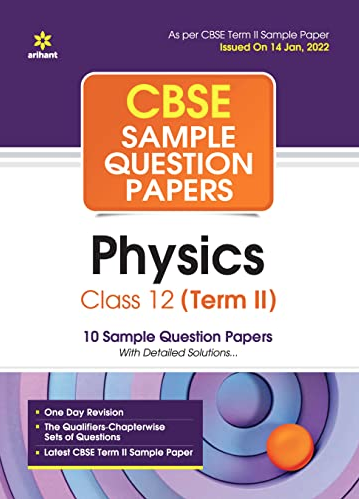# Light of wavelength 2000 Å falls on a metal surface of work function 4.2 eV. (a) What is the kinetic energy (in eV) of the fastest electrons emitted from the surface? (b) What will be the change in the energy of the emitted electrons if the intensity of light with same wavelength is doubled? (c) If the same light falls on another surface of work function 6.5 eV, what will be the energy of emitted electrons?

CBSE Sample Question Paper, Class 12 Physics Term 2 Question - Light of wavelength 2000 Å falls on a metal surface of work function 4.2 eV. (a) What is the kinetic energy (in eV) of the fastest electrons emitted from the surface? (b) What will be the change in the energy of the emitted electrons if the intensity of light with same wavelength is doubled? (c) If the same light falls on another surface of work function 6.5 eV, what will be the energy of emitted electrons?